Courses

# Quantitative Aptitude - Test 3

## 25 Questions MCQ Test SSC CGL Tier 1 Mock Test Series | Quantitative Aptitude - Test 3

Description
This mock test of Quantitative Aptitude - Test 3 for SSC helps you for every SSC entrance exam. This contains 25 Multiple Choice Questions for SSC Quantitative Aptitude - Test 3 (mcq) to study with solutions a complete question bank. The solved questions answers in this Quantitative Aptitude - Test 3 quiz give you a good mix of easy questions and tough questions. SSC students definitely take this Quantitative Aptitude - Test 3 exercise for a better result in the exam. You can find other Quantitative Aptitude - Test 3 extra questions, long questions & short questions for SSC on EduRev as well by searching above.
QUESTION: 1

### Which of the following numbers is the smallest?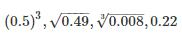Solution:

Before comparing 1st we will try to bring the given numbers to their simplest form.
(0.5)3 = 0.125
√0.49 = √ (0.7)2 = 0.7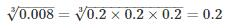0.22
Now, comparing the 4 numbers we can easily say, 1stone is the smallest.
∴ The smallest number is (0.5)3 = 0.125

QUESTION: 2

###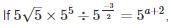then a is equal to

Solution:

Before we solve this completely, we simplify the LHS using BODMAS rule, and a few rules from indices.
First, we express every quantity in terms of powers of 5.
Hence,
5√5 = 53/2
Hence, we have
53/2 × 5÷ 5-3/2 = 5a+2
Now here, we use BODMAS rule. It is described as follows:
B – Brackets
O – Of
D – Division
M – Multiplication
S – Subtraction
The above is the order in which the operations are to be solved. We observe that there are no brackets or “Of” in the given expression. Hence, we go ahead with division.
5÷ 5-3/2 = 55+3/2 = 513/2
Now, we have multiplication
⇒ 53/2 × 513/2 = 516/2 = 58
Equating the above with RHS,
5= 5a+2
⇒ 8 = a + 2
⇒ a = 6

QUESTION: 3

### The number of students in a class is increased by 20% and the number now becomes 66. Initially the number was

Solution:

Let the initial number of students be ‘n’.
Given, number of students in a class is increased by 20% and the number now becomes 66
n + 20% of n = 66
⇒ 1.2n = 66
⇒ n = 55

QUESTION: 4

The price of a jewel varies as the square of its weight. Two jewels A and B whose weights are in the ratio of 3 and 5 are combined and made a new jewel C. What is the ratio of price of C to the combined price of A and B?

Solution:

Suppose the weight of the jewel is W and its price is P then according to the question
P ∝ W2,
⇒ P = kW2    where K is the constant.
The ratio between the weights of A and B is 3 : 5. So let’s assume the weight of A is 3x and weight of B is 5x.
So, Price of A = k(3x)2 = 9kx2
And price of B = k(5x)2 = 25kx2
Now, weight of C = weight of A + weight of B = 3x + 5x = 8x
So, price of C = k(8x)2 = 64kx2
Combined price of A and B = 9kx2 + 25kx= 34kx2
So the required ratio = 64kx2 : 34kx2 = 32 : 17.

QUESTION: 5

4 litres of a 40% solution of spirit in water is mixed with 6 litres of a 50% solution of spirit in water. What is the strength of spirit in the resulting mixture?

Solution:

Quantity of spirit in 4 litres solution with 40% concentration = (40/100) × 4 = 1.6 litres
Quantity of spirit in 6 litres solution with 50% concentration = (50/100) × 6 = 3.0 litres
Thus, amount of spirit in resulting mixture = 1.6 + 3.0 = 4.6 litres
Total amount of resulting mixture = 4 + 6 = 10 litres
Strength of spirit in resulting mixture = (4.6/10) × 100 = 46%

QUESTION: 6

If LCM of two numbers is thrice the larger number and difference between the smaller number and the HCF is 14. Then, find out the smaller number of the two numbers.

Solution:

Solution: Let the larger number be x and the smaller number be y.
It is given that LCM is thrice of the larger number,
Therefore, LCM = 3x      ----(i)
It is also given that difference between the smaller number and the HCF is 14,
Therefore, y – HCF = 14
HCM = y – 14      ----(ii)
We know that product of two numbers = LCM × HCF
Therefore, x × y = 3x × (y – 14)      (from (i) and (ii))
y = 3(y – 14)
y = 3y – 42
2y = 42
y = 21
Hence, the smaller number is 21.

QUESTION: 7

The ratio of ages of A and B is 11 : 13 respectively. After 7 years the ratio of their ages will be 20 : 23. What is the difference between their ages?

Solution:

Let the ages of A and B be 11x and 13x respectively. Then,
According to the question, after 7 years the ratio of their ages will be 20 : 23.
⇒ (11x + 7)/(13x + 7) = 20/23
⇒ 260x + 140 = 253x + 161
⇒ 260x – 253x = 161 – 140
⇒ 7x = 21
⇒ x = 3
∴ The difference between their ages = 13x – 11x = 2x = 2 × 3 = 6 years
Hence, the required difference between the ages of A and B is 6 years.

QUESTION: 8

The value of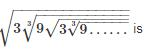Solution: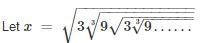On Squaring we get,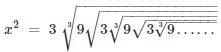On Cubing we get,
x= 27 × 9x
⇒ x5 = 243
⇒ x = 3

QUESTION: 9

By selling cloth at Rs. 9 per metre, a shopkeeper loses 10%. Find the rate at which it should be sold so as to earn profit of 15%​

Solution:

Let the CP of 1 m cloth = Rs x
Loss% = 10%
SP of 1 m cloth = Rs 9
Therefore,
Loss = x – 9
10 = ((x – 9)/x × 100%
x – 9 = x/10
x = 10
now CP of 1 m cloth = Rs 10
let the selling price be Rs y
profit% = 15% = ((y – 10)/10 × 100%)
3 = 2(y – 10)
2y – 20 = 3
y = 23/2
y = 11.5
SP of 1 m cloth = Rs. 11.50

QUESTION: 10

Rs. 3,000 is divided among A, B and C, so that A receives 1/3 as much as B and C together receive and B receives 2/3 as much as A and C together receive. Then share of C is

Solution:

Given, 3,000 is divided among A, B and C, so that A receives 1/3 as much as B and C together receive and B receives 2/3 as much as A and C together receive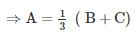⇒ 3A = B + C……….(1)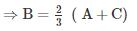⇒ 3B = 2A + 2C…………(2)
⇒ Putting the value of C from (1) into (2), we get,
⇒ 3B = 2 A + 2 (3A – B)
⇒ 8A = 5B …………….(3)
⇒ Similarly, we can solve and will get relation between B and C.
⇒ 7B = 8C …………….. (4)
⇒ Multiplying (3) with 7 and (4) with 5, we get,
⇒ 56A = 35B = 40C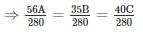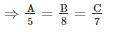⇒ A : B : C = 5 : 8: 7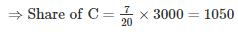QUESTION: 11

At some rate per annum the amount with compound interest on Rs. 1600 for 2 years is Rs. 1730.56. The rate of interest per annum is:

Solution:

We know that,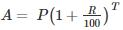Where, A = Final amount, P = Principal amount, R = % rate of interest, T = time period in years
Here, P = Rs. 1600, T = 2 years, A = 1730.56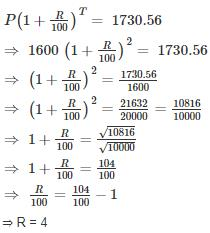Thus, rate of R% per annum is 4%

QUESTION: 12

I walk a certain distance and ride back taking a total time of 37 minutes. I could walk both ways in 55 minutes. How long would it take me to ride both ways?

Solution:

Speed = distance/time
Let the distance be x km. Then,
(Time taken to walk x km) + (Time taken to ride x km) = 37 min.
⇒ (Time taken to walk 2x km) + (Time taken to ride 2x km) = 74 min.
Given, time taken to walk 2x km = 55 min.
Time taken to ride 2x km = (74 - 55) min = 19 min

QUESTION: 13

Two trains start at the same time from two point P and Q and proceed towards each other at the speed of 75 km/hr and 100 km/hr respectively. When they meet, it is found that first train has travelled 125 km less than the second train. Find the distance between point P and Q.

Solution:

Let the distance travelled by first train till the point they meet be X km. Since the second train travelled 125 Km more than the first one, thus
Distance travelled by second train when they meet = X + 125
We know that, Time = Distance/Speed
We know that both trains travelled for the same time till they meet. Hence,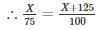⇒ 100X = 75X + 9375
⇒ X = 375 Km
∴ X + 125 = 500 Km
Hence the distance between the points P and Q = Distance travelled by train A & B
= 375 + 500
= 875 Km

QUESTION: 14

Aman and Akbar together can do a work in 18 days. Both of them began to work. After 4 days Akbar fell ill, Aman completed the remaining work in 42 days. In how many days can Aman complete the whole work if he did it alone?

Solution:

Let the total work be W units.
Amount of work done by Aman in one day = Am units
Amount of work done by Akbar in one day = Ak units
W = (Am + Ak) 18
Now this piece of work has been started, but both worked for only 4 days, after which Aman took over,
⇒ W = (Am + Ak) 4 + (Am) 42
⇒ (Am + Ak) 18 = (Am + Ak) 4 + (Am)42
⇒ (Am + Ak) 14 = (Am) 42
⇒ Am + Ak = 3 Am
⇒ Ak = 2 Am
Substituting the value of Ak in the very first equation, we have
W = (Am + 2 Am)18
⇒ W = 54 Am
Hence, Aman alone would have taken 54 days to complete the work.

QUESTION: 15

The sides of a right-angled triangle forming right angle are in the ratio 5 : 12. If the area of the triangle is 270 cm2, then the length of the hypotenuse is

Solution:

Given data-
Sides of a right-angled triangle forming right angle are in the ratio 5 : 12
Let sides of triangle forming right angle be 5x & 12x respectively, where x is constant of proportionality.
Area of the triangle = ½ × base × height = ½ × 5x × 12x = 30x2
30x2 = 270
⇒ x2 = 9
⇒ x = 3
∴ sides of triangle forming right angle are-
5x = 5 × 3 = 15 cm
& 12x = 12 × 3 = 36 cm
Using Pythagoras theorem
hypotenuse2 = 152 + 362
⇒ hypotenuse2 = 225 + 1296
⇒ hypotenuse2 = 1521
∴ Hypotenuse = √1521 = 39 cm

QUESTION: 16

Compute the ratio of the volumes of two cylinders if the ratio of radii of the bases is 9 : 4 and the ratio of their heights is 3 : 7

Solution:

Volume of a cylinder = πr2 × h
Hence the ratio of their volumes
= (πr12 × h1)/(πr22 × h2)
= (92 × 3)/(42 × 7)
= 243/112

QUESTION: 17

AB and CD are two parallel chords on the opposite sides of the centre of the circle. If AB = 18 cm, CD = 24 cm and the radius of the circle is 15 cm, the distance between the chords is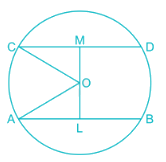Solution:

Perpendiculars drawn from the centre on the chord bisects them.
Given, AB and CD are two parallel chords on the opposite sides of the centre of the circle.
AB = 18 cm, CD = 24 cm and the radius of the circle is 15 cm
From the figure,
OM and OL are perpendiculars, OC and OA are radius
∴ CM = CD/2 = 12 cm and AL = AB/2 = 9 cm
By Pythagoras theorem:
OC2 = OM2 + MC2
⇒ OM2 = 152 – 122 = 81
⇒ OM = 9 cm
Similarly,
OA2 = OL2 + AL2
⇒ OL2 = 152 – 92 = 144
⇒ OL = 12 cm
Distance between the two chords = OL + OM
⇒ Distance between the two chord = 12 + 9 cm = 21 cm

QUESTION: 18

Two circles intersect at A and B. P is a point on produced BA. PT and PQ are tangents to the circles. The relation of PT and PQ is

Solution: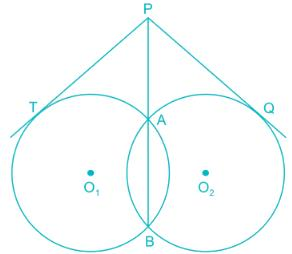We know that,
Property- If MNO is a secant to a circle intersecting the circle at M and N and OT is a tangent segment, then
OM × ON = OT2
From the diagram,
PAB is a secant to a circle with center O1 intersecting the circle at A and B and PT is a tangent segment,
∴ PA × PB = PT2  ……. (1)
Again, PAB is a secant to a circle with center O2 intersecting the circle at A and B and PQ is a tangent segment,
∴ PA × PB = PQ2  ……. (2)
From (1) and (2)
PT2 = PQ2
⇒ PT = PQ

QUESTION: 19

If XYZ is an equilateral triangle and S is a point on YZ such that XS ⊥ YZ, then

Solution:

As we know, in an equilateral triangle all sides are equal.
∴ XY = YZ = ZX
Let XY = 2a units
∴ YZ = ZX = 2a units
And XS is perpendicular bisector of side YZ
∴ YS = SZ = a units
Thus, XY: YS = 2: 1

QUESTION: 20

ABCD is a cyclic trapezium whose sides AD and BC are parallel to each other. If ∠ABC = 72°, then the measure of the ∠BCD is

Solution: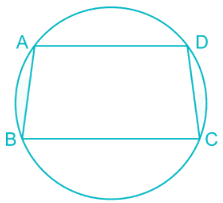As stated in above figure ABCD is a cyclic trapezium with Line AD and Line BC parallel to each other.
As per the properties of cyclic quadrilateral,
Also, Properties of parallel lines
∠BCD = 180° - ∠ADC = 180° - 108° = 72°
∴ ∠ABC = ∠BCD
⇒ ∠BCD= 72°

QUESTION: 21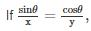sinθx=cosθy,then sinθ + cos θ is equal to

Solution: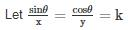∴ sinθ = kx and cosθ = ky
sin2θ + cos2θ = 1
⇒ k2x2 + k2y2 = 1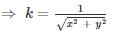sinθ + cos θ = kx + ky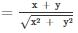QUESTION: 22

The angle of elevation of the top a tower form the base of a building is 60° and the angle of elevation of the top of the building from the base of the tower is 30°. If the height of tower is 125 m, find the height of the building.

Solution: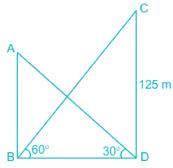Consider AB as height of building and CD be as height of tower
AB=?
From trigonometric formulas,
Tan θ = perpendicular/base
∴ in ΔBCD
tan 60° = CD/BD
⇒ √3 = CD/BD
⇒ 125/√ 3 = BD
Also, in ΔABD
tan 30° = AB/BD
⇒ 125/√ (3 × 3) = AB
⇒ AB = 125/3 = 41.66m

QUESTION: 23

The value of 2cos220° + 3cos270° + sin270° is

Solution:

2cos220° + 3cos270° + sin270°
= 2cos220° + 2cos270° + cos270° + sin270°
= 2cos220° + 2cos(90 – 20)° + 1
= 2cos220° + 2sin220° + 1
= 2 + 1 = 3

QUESTION: 24

5 years ago the average age of a family of 3 members was 19 years. A baby having been born, the average age of the family remains the same today. The age of the baby after 8 years would be

Solution:

According to the given information,
5 years ago the average age of a family of 3 members was 19 years.
(x – 15)/3 = 19
⇒ x = 72
A baby having been born, the average age of the family remains the same i.e. 19 years
Let the present age of baby be ‘b’ years
⇒ (72+b)/4 = 19
⇒ b = 4
The age of the baby after 8 years would be 4 + 8 = 12 years

QUESTION: 25

The sum of all odd numbers between 11 and 61 is

Solution:

Using Sum of Arithmetic Progression
Total odd numbers between 11 and 61, n = 24
Sum = n/2[a + l] = 24/2[13 + 59] (a = first term, l = last term)
= 24/2 × 72
= 864